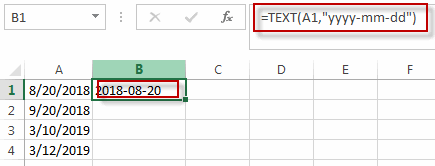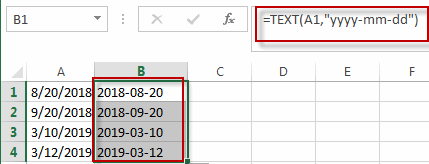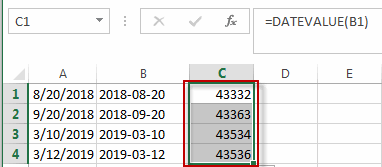# Convert Date to Text

This post will guide you how to convert dates stored as text format in Excel. How do I convert the date as date format to text format in excel. You can use a variety of formulas to convert the dates in your worksheet to text format.

You can use the TEXT function to create a formula to convert the date as date format to text format.

Assuming that you have a list of data that contain date values and you want to convert its format as text format. Just do the following steps:

#1 type the following formula into a blank cell.

=TEXT(B1,”yyyy-mm-dd”), press Enter key in your keyboard#2 drag the AutoFill Handle over other cells to apply this formula.If you want to convert a date as Text format to number, you can use the DATEVALUE function or just use the Format Cell function to achieve it. Such as: =DATEVALUE(“2018/23/08”)### Related Functions

• Excel Text function
The Excel TEXT function converts a numeric value into text string with a specified format. The TEXT function is a build-in function in Microsoft Excel and it is categorized as a Text Function. The syntax of the TEXT function is as below: = TEXT (value, Format code)…
• Excel DATEVALUE Function
The Excel DATEVALUE function returns the serial number of date. And it can be used to convert a date represented as text format into a serial number that recognizes as a date format.The syntax of the DATEVALUE function is as below:=DATEVALUE(date_text)…